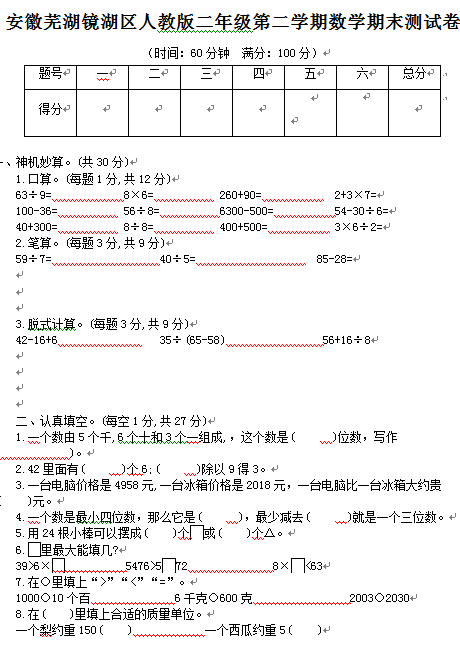（时间：60分钟  满分：100分）

1.口算。(每题1分,共12分)

63÷9=　　　　　　8×6=　　　　　 260+90=　　　　　  2+3×7=

100-36=　　　　　 56÷8=　　　　　6300-500=　　　　　54-30÷6=

40+300=　　　　　 8÷8=　　　　　 400+500=　　　　　 3×6÷2=

2.笔算。(每题3分,共9分)

59÷7=　　　　　　　　　40÷5=　　　　　　　　　  85-28=

【DOC文档4页】安徽芜湖镜湖区人教版小学二年级第二学期数学期末测试卷含答案A4电子版资料_可直接打印_会员免费下载##### 评论信息